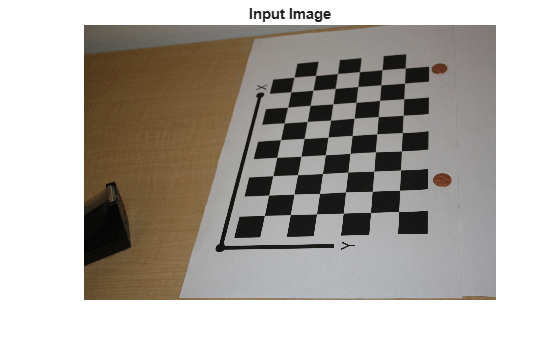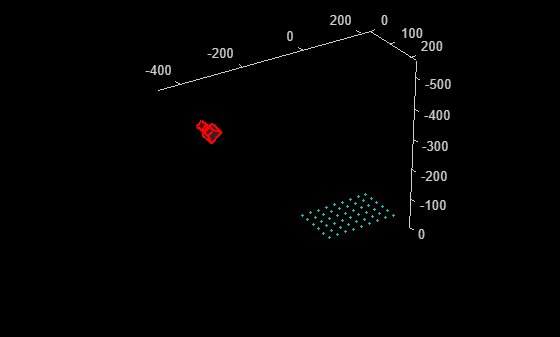# estimateExtrinsics

Calculate location of calibrated camera

## Syntax

``````camExtrinsics = estimateExtrinsics(imagePoints,worldPoints,intrinsics)``````

## Description

example

``````camExtrinsics = estimateExtrinsics(imagePoints,worldPoints,intrinsics)``` returns the camera extrinsics, which are 3-D rigid transformation that enables you to transform points from the world coordinate to the camera coordinate system.```

## Examples

collapse all

Create a set of calibration images.

```images = imageDatastore(fullfile(toolboxdir("vision"),"visiondata", ... "calibration","slr"));```

Detect the checkerboard corners in the images.

`[imagePoints,boardSize] = detectCheckerboardPoints(images.Files);`

Generate the world coordinates of the checkerboard corners in the pattern-centric coordinate system, with the upper-left corner at (0,0). The square size is in millimeters.

```squareSize = 29; worldPoints = generateCheckerboardPoints(boardSize,squareSize);```

Calibrate the camera.

```I = readimage(images,1); imageSize = [size(I,1) size(I,2)]; cameraParams = estimateCameraParameters(imagePoints,worldPoints, ... ImageSize=imageSize); intrinsics = cameraParams.Intrinsics;```

Load an image at a new location.

```imOrig = readimage(images,9); figure imshow(imOrig) title("Input Image")```Undistort the image.

`[im,newOrigin] = undistortImage(imOrig,intrinsics,OutputView="full");`

Find the reference object in the new image.

`[imagePoints,boardSize] = detectCheckerboardPoints(im);`

Compensate for the image coordinate system shift.

`imagePoints = imagePoints+newOrigin;`

Calculate new extrinsics.

`camExtrinsics = estimateExtrinsics(imagePoints,worldPoints,intrinsics);`

Calculate the camera pose.

```camPose = extr2pose(camExtrinsics); figure plotCamera(AbsolutePose=camPose,Size=20); hold on pcshow([worldPoints,zeros(size(worldPoints,1),1)], ... VerticalAxisDir="down",MarkerSize=40);```## Input Arguments

collapse all

Image coordinates of points, specified as an M-by-2 array. The array contains M number of [x, y] coordinates. The `imagePoints` and `worldPoints` inputs must both be `double` or both be `single`.

Data Types: `single` | `double`

World coordinates corresponding to image coordinates, specified as an M-by-2 matrix. The `imagePoints` and `worldPoints` inputs must both be `double` or both be `single`. The function assumes that the points are coplanar with z = 0 and the number of points, M, must be at least 4.

Data Types: `single` | `double`

Camera intrinsics, specified as a `cameraIntrinsics` or a `fisheyeIntrinsics` object. The object stores information about a camera’s intrinsic calibration parameters, including the lens distortion parameters.

## Output Arguments

collapse all

Camera extrinsics, returned as a `rigidtform3d` object. The `"R"` and the `"Translation"` properties of the object represent the orientation and location of the camera.

## Algorithms

The `estimateExtrinsics` function uses two different algorithms to compute the extrinsics depending on whether `worldPoints` are specified as an M-by-2 matrix. Use an M-by-2 matrix for coplanar points where z= 0.

The `estimateExtrinsics` function computes the rotation matrix and translation vector for a single image in closed form. During calibration, the extrinsics are estimated numerically to minimize the reprojection errors for all calibration images. Therefore, using the `estimateExtrinsics` function on one of the calibration images returns rotation matrix and translation vector slightly different from the ones obtained during calibration.

## Version History

Introduced in R2022b

expand all